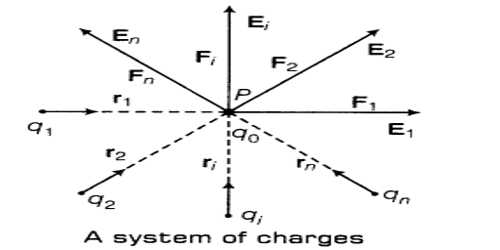Physics

# Superposition Principle of Electric ForceSuperposition principle of electric force

In order to find the resultant force, all charges are considered separately with that particular charge as if all other charges are absent. In this way, for each charge, the force on that particular charge is to be found out. Then the vector sum of all the forces gives the resultant force on that charge. It is called the superposition principle of electric force.

Explanation

Let three positive charges q1, q2, and q3 be located at a close distance [Figure]. Now, the repulsive force on q3 due to charges q1 and q2 is to be found out. First, let us find both the magnitude and direction of the force F31 acting on charge q3 due to charge q11. Similarly, magnitude and direction of the force F32 acting on q3 due to q2 are found out. Now the resultant force F3 will be the vector sum of F31 and F32, i.e., F3 = F31 + F32.It is to be noted that while finding the force on q3 due to q1, charge q2 has been considered to be absent. Similarly, for calculating the force on q3 due to q2, q1 has been considered to be absent. Following this procedure, we can find the net force acting on a charge due to any number of surrounding charges.